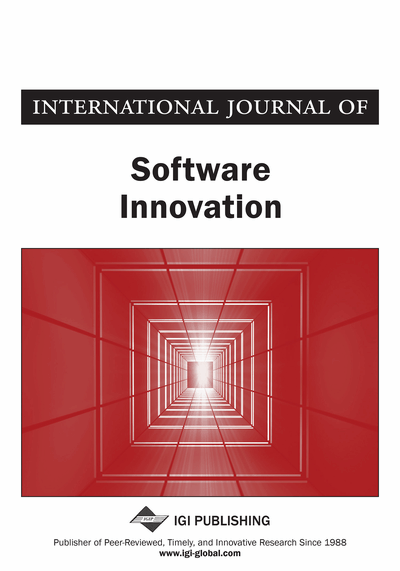# A Source Code Plagiarism Detecting Method Using Sequence Alignment with Abstract Syntax Tree Elements

Hiroshi Kikuchi (Graduate School of Informatics and Engineering, The University of Electro-Communications, Chofu, Japan), Takaaki Goto (Graduate School of Informatics and Engineering, The University of Electro-Communications, Chofu, Japan), Mitsuo Wakatsuki (Graduate School of Informatics and Engineering, The University of Electro-Communications, Chofu, Japan) and Tetsuro Nishino (Graduate School of Informatics and Engineering, The University of Electro-Communications, Chofu, Japan)
DOI: 10.4018/IJSI.2015070104
Available
\$37.50
No Current Special Offers

## Abstract

Learning to program is an important subject in computer science courses. During programming exercises, plagiarism by copying and pasting can lead to problems for fair evaluation. Some methods of plagiarism detection are currently available, such as sim. However, because sim is easily influenced by changing the identifier or program statement order, it fails to do enough to support plagiarism detection. In this paper, the authors propose a plagiarism detection method which is not influenced by changing the identifier or program statement order. The authors also explain our method's capabilities by comparing it to the sim plagiarism detector. Furthermore, the authors reveal how our method successfully detects the presence of plagiarism.
Article Preview
Top

## 2. Background

### 2.1. Sequence Alignment

Sequence alignment is a method to calculate a correspondence relationship among strings by adding a space or shifting the alphabetic positions. Strings obtained after alignment are also called sequence alignment. The similarity between two strings can be described by a score. In our method, we first obtain tokens by lexical analysis, and then calculate the sequence alignment and compare the obtained similarity scores.

Here is an example. Let s and t be strings. Sometimes the lengths of s and t are different. We insert a gap symbol “-” in order to line up the strings’ lengths. This process is called alignment. Figure 1 illustrates an example of calculating the alignment between “masters” and “stars”.

As described in Figure 1, it is possible to have several patterns of alignment. Usually, sequence alignment includes a minimum number of gap symbols and fewer different characters in the two strings. After obtaining the alignment, we then compare each character in the same position in the two strings. If two characters are the same, we score it as m point. If two characters are different, we score it as d. Moreover, if one or both characters include a gap symbol, then we score it as g. We can obtain an alignment score by adding the scores of every character. If m ≥ d ≥ g or m ≥ g ≥ d, the alignment with the maximum number of total scores is the optimal alignment. If we assume m = 1; d = −1; g = −2, then we can obtain alignment scores for the left- and right-hand sides of Figure 1 as −6 and −1, respectively. It can be seen that the right-hand alignment is appropriate for this example.

Usually, calculating an alignment score can be done using dynamic programming. The Needleman-Wunsch algorithm (Needleman, 1970) is an efficient algorithm based on dynamic programming.

Calculating the alignment score by using dynamic programming is done as follows (Akutsu, 2007). Let Σ be a set of characters. Let s and t be strings, andandindicate the length of each string. s[i] means the ith character in a string. We also use “−” as a gap symbol. Note that the gap symbol is not a member of Σ, and it cannot appear in the same position of the alignments.

Here we show a definition of score function which calculates scores by checking consistent and inconsistent gaps for each character in the strings.is a function which means

,it holdsunder. In this paper, we use the following definition:(1) where

.

Let D be a matrix of. Matrix D stores the intermediate results of the alignment scores, which can be calculated by the following recursive formula:

(2)(3)(4)

This formula calculates the maximum weighted path on the alignment graph as shown in Figure 2.

## Complete Article List

Search this Journal:
Reset
Open Access Articles
Volume 10: 4 Issues (2022): Forthcoming, Available for Pre-Order
Volume 9: 4 Issues (2021): 3 Released, 1 Forthcoming
Volume 8: 4 Issues (2020)
Volume 7: 4 Issues (2019)
Volume 6: 4 Issues (2018)
Volume 5: 4 Issues (2017)
Volume 4: 4 Issues (2016)
Volume 3: 4 Issues (2015)
Volume 2: 4 Issues (2014)
Volume 1: 4 Issues (2013)
View Complete Journal Contents Listing